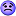View Single PostLhancelot
 10.15.2019 , 09:01 AM | #12 Quote Quote: Originally Posted by dipstikhere are results for gcd casts. would be nice to have this data for channels (diagnostic scan/ lightning strike) using data from https://docs.google.com/spreadsheets...gid=1526455495 summary: https://pasteboard.co/IzZn0PG.png Two-Sample T-Test and CI: 0, 862 Two-sample T for 0 vs 862 N Mean StDev SE Mean 0 20 1.5465 0.0500 0.011 862 20 1.4988 0.0170 0.0038 Difference = mu (0) - mu (862) Estimate for difference: 0.0478 95% CI for difference: (0.0233, 0.0722) T-Test of difference = 0 (vs not =): T-Value = 4.04 P-Value = 0.001 DF = 23 Boxplot of 0, 862 Two-Sample T-Test and CI: 0, 755 Two-sample T for 0 vs 755 N Mean StDev SE Mean 0 20 1.5465 0.0500 0.011 755 21 1.50029 0.00901 0.0020 Difference = mu (0) - mu (755) Estimate for difference: 0.0463 95% CI for difference: (0.0226, 0.0699) T-Test of difference = 0 (vs not =): T-Value = 4.08 P-Value = 0.001 DF = 20 Boxplot of 0, 755 Two-Sample T-Test and CI: 0, 1293 Two-sample T for 0 vs 1293 N Mean StDev SE Mean 0 20 1.5465 0.0500 0.011 1293 22 1.4120 0.0366 0.0078 Difference = mu (0) - mu (1293) Estimate for difference: 0.1346 95% CI for difference: (0.1069, 0.1623) T-Test of difference = 0 (vs not =): T-Value = 9.88 P-Value = 0.000 DF = 34 Two-Sample T-Test and CI: 0, 1294 Two-sample T for 0 vs 1294 N Mean StDev SE Mean 0 20 1.5465 0.0500 0.011 1294 22 1.4133 0.0341 0.0073 Difference = mu (0) - mu (1294) Estimate for difference: 0.1333 95% CI for difference: (0.1062, 0.1604) T-Test of difference = 0 (vs not =): T-Value = 10.00 P-Value = 0.000 DF = 33 Two-sample T for 0 vs 1724 N Mean StDev SE Mean 0 20 1.5465 0.0500 0.011 1724 22 1.4030 0.0213 0.0045 Difference = mu (0) - mu (1724) Estimate for difference: 0.1435 95% CI for difference: (0.1187, 0.1683) T-Test of difference = 0 (vs not =): T-Value = 11.90 P-Value = 0.000 DF = 25 Two-sample T for 0 vs 2155 N Mean StDev SE Mean 0 20 1.5465 0.0500 0.011 2155 22 1.4012 0.0222 0.0047 Difference = mu (0) - mu (2155) Estimate for difference: 0.1453 95% CI for difference: (0.1203, 0.1703) T-Test of difference = 0 (vs not =): T-Value = 11.97 P-Value = 0.000 DF = 25 Two-sample T for 862 vs 755 N Mean StDev SE Mean 862 20 1.4988 0.0170 0.0038 755 21 1.50029 0.00901 0.0020 Difference = mu (862) - mu (755) Estimate for difference: -0.00149 95% CI for difference: (-0.01027, 0.00730) T-Test of difference = 0 (vs not =): T-Value = -0.35 P-Value = 0.732 DF = 28 Two-sample T for 862 vs 1293 N Mean StDev SE Mean 862 20 1.4988 0.0170 0.0038 1293 22 1.4120 0.0366 0.0078 Difference = mu (862) - mu (1293) Estimate for difference: 0.08685 95% CI for difference: (0.06912, 0.10457) T-Test of difference = 0 (vs not =): T-Value = 10.01 P-Value = 0.000 DF = 30 Two-sample T for 862 vs 1724 N Mean StDev SE Mean 862 20 1.4988 0.0170 0.0038 1724 22 1.4030 0.0213 0.0045 Difference = mu (862) - mu (1724) Estimate for difference: 0.09575 95% CI for difference: (0.08378, 0.10773) T-Test of difference = 0 (vs not =): T-Value = 16.17 P-Value = 0.000 DF = 39 Two-sample T for 862 vs 2155 N Mean StDev SE Mean 862 20 1.4988 0.0170 0.0038 2155 22 1.4012 0.0222 0.0047 Difference = mu (862) - mu (2155) Estimate for difference: 0.09757 95% CI for difference: (0.08527, 0.10988) T-Test of difference = 0 (vs not =): T-Value = 16.05 P-Value = 0.000 DF = 38 Two-sample T for 862 vs 3017 N Mean StDev SE Mean 862 20 1.4988 0.0170 0.0038 3017 22 1.3983 0.0139 0.0030 Difference = mu (862) - mu (3017) Estimate for difference: 0.10048 95% CI for difference: (0.09070, 0.11027) T-Test of difference = 0 (vs not =): T-Value = 20.82 P-Value = 0.000 DF = 36 Two-sample T for 1293 vs 3017 N Mean StDev SE Mean 1293 22 1.4120 0.0366 0.0078 3017 22 1.3983 0.0139 0.0030 Difference = mu (1293) - mu (3017) Estimate for difference: 0.01364 95% CI for difference: (-0.00350, 0.03078) T-Test of difference = 0 (vs not =): T-Value = 1.64 P-Value = 0.114 DF = 26 Two-sample T for 1724 vs 3017 N Mean StDev SE Mean 1724 22 1.4030 0.0213 0.0045 3017 22 1.3983 0.0139 0.0030 Difference = mu (1724) - mu (3017) Estimate for difference: 0.00473 95% CI for difference: (-0.00625, 0.01571) T-Test of difference = 0 (vs not =): T-Value = 0.87 P-Value = 0.388 DF = 36 Two-sample T for 2155 vs 3017 N Mean StDev SE Mean 2155 22 1.4012 0.0222 0.0047 3017 22 1.3983 0.0139 0.0030 Difference = mu (2155) - mu (3017) Estimate for difference: 0.00291 95% CI for difference: (-0.00843, 0.01425) T-Test of difference = 0 (vs not =): T-Value = 0.52 P-Value = 0.606 DF = 35 Two-sample T for 2155 vs 3448 N Mean StDev SE Mean 2155 22 1.4012 0.0222 0.0047 3448 23 1.3080 0.0270 0.0056 Difference = mu (2155) - mu (3448) Estimate for difference: 0.09327 95% CI for difference: (0.07843, 0.10811) T-Test of difference = 0 (vs not =): T-Value = 12.68 P-Value = 0.000 DF = 42 I am sure this is a lot of important information but sadly it looks like an alien life form wrote it out to me. That or maybe Einstein, lol. I wish mathematical analytics was my strong point.BIOWARE Players quit WZs they do not enjoy. You are losing PVPers. Give players a choice of WZs, enough is enough (Huttball) already! Good job increasing rewards for PVP! It was much needed, thank you.